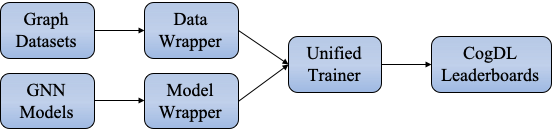# Model Training

## Introduction to graph representation learning

Inspired by recent trends of representation learning on computer vision and natural language processing, graph representation learning is proposed as an efficient technique to address this issue. Graph representation aims at either learning low-dimensional continuous vectors for vertices/graphs while preserving intrinsic graph properties, or using graph encoders to an end-to-end training.

Recently, graph neural networks (GNNs) have been proposed and have achieved impressive performance in semi-supervised representation learning. Graph Convolution Networks (GCNs) proposes a convolutional architecture via a localized first-order approximation of spectral graph convolutions. GraphSAGE is a general inductive framework that leverages node features to generate node embeddings for previously unseen samples. Graph Attention Networks (GATs) utilizes the multi-head self-attention mechanism and enables (implicitly) specifying different weights to different nodes in a neighborhood.

## Unified Trainer

CogDL provides a unified trainer for GNN models, which takes over the entire loop of the training process. The unified trainer, which contains much engineering code, is implemented flexibly to cover arbitrary GNN training settings.We design four decoupled modules for the GNN training, including Model, Model Wrapper, Dataset, Data Wrapper. The Model Wrapper is for the training and testing steps, while the Data Wrapper is designed to construct data loaders used by Model Wrapper.

The main contributions of most GNN papers mainly lie on three modules except Dataset, as shown in the table. For example, the GCN paper trains the GCN model under the (semi-)supervised and full-graph setting, while the DGI paper trains the GCN model by maximizing local-global mutual information. The training method of the DGI is considered as a model wrapper named dgi_mw, which could be used for other scenarios.

Paper

Model

Model Wrapper

Data Wrapper

GCN

GCN

supervised

full-graph

GAT

GAT

supervised

full-graph

GraphSAGE

SAGE

sage_mw

neighbor sampling

Cluster-GCN

GCN

supervised

graph clustering

DGI

GCN

dgi_mw

full-graph

Based on the design of the unified trainer and decoupled modules, we could do arbitrary combinations of models, model wrappers, and data wrappers. For example, if we want to apply DGI to large-scale datasets, all we need is to substitute the full-graph data wrapper with the neighbor-sampling or clustering data wrappers without additional modifications. If we propose a new GNN model, all we need is to write essential PyTorch-style code for the model. The rest could be automatically handled by CogDL by specifying the model wrapper and the data wrapper. We could quickly conduct experiments for the model using the trainer via textit{trainer = Trainer(epochs,…)} and textit{trainer.run(…)}. Moreover, based on the unified trainer, CogDL provides native support for many useful features, including hyperparameter optimization, efficient training techniques, and experiment management without any modification to the model implementation.

## Experiment API

CogDL provides a more easy-to-use API upon Trainer, i.e., experiment. We take node classification as an example and show how to use CogDL to finish a workflow using GNN. In supervised setting, node classification aims to predict the ground truth label for each node. CogDL provides abundant of common benchmark datasets and GNN models. On the one hand, you can simply start a running using models and datasets in CogDL. This is convenient when you want to test the reproducibility of proposed GNN or get baseline results in different datasets.

```from cogdl import experiment
experiment(model="gcn", dataset="cora")
```

Or you can create each component separately and manually run the process using `build_dataset`, `build_model` in CogDL.

```from cogdl import experiment
from cogdl.datasets import build_dataset
from cogdl.models import build_model
from cogdl.options import get_default_args

args = get_default_args(model="gcn", dataset="cora")
dataset = build_dataset(args)
model = build_model(args)
experiment(model=model, dataset=dataset)
```

As show above, model/dataset are key components in establishing a training process. In fact, CogDL also supports customized model and datasets. This will be introduced in next chapter. In the following we will briefly show the details of each component.

## How to save trained model?

CogDL supports saving the trained model with `checkpoint_path` in command line or API usage. For example:

```experiment(model="gcn", dataset="cora", checkpoint_path="gcn_cora.pt")
```

When the training stops, the model will be saved in gcn_cora.pt. If you want to continue the training from previous checkpoint with different parameters(such as learning rate, weight decay and etc.), keep the same model parameters (such as hidden size, model layers) and do it as follows:

```experiment(model="gcn", dataset="cora", checkpoint_path="gcn_cora.pt", resume_training=True)
```

In command line usage, the same results can be achieved with `--checkpoint-path {path}` and `--resume-training`.

## How to save embeddings?

Graph representation learning (network embedding and unsupervised GNNs) aims to get node representation. The embeddings can be used in various downstream applications. CogDL will save node embeddings in the given path specified by `--save-emb-path {path}`.

```experiment(model="prone", dataset="blogcatalog", save_emb_path="./embeddings/prone_blog.npy")
```

Evaluation on node classification will run as the end of training. We follow the same experimental settings used in DeepWalk, Node2Vec and ProNE. We randomly sample different percentages of labeled nodes for training a liblinear classifier and use the remaining for testing We repeat the training for several times and report the average Micro-F1. By default, CogDL samples 90% labeled nodes for training for one time. You are expected to change the setting with `--num-shuffle` and `--training-percents` to your needs.

In addition, CogDL supports evaluating node embeddings without training in different evaluation settings. The following code snippet evaluates the embedding we get above:

```experiment(
model="prone",
dataset="blogcatalog",
```# Get embedding